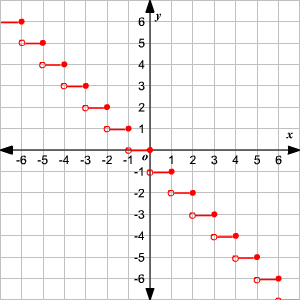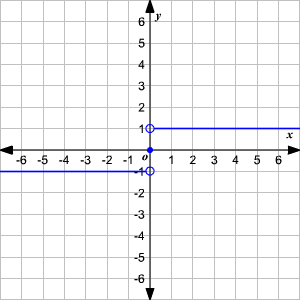# Step Functions

A step function is a piecewise-defined function in which every piece is a horizontal line segment or a point.

Example 1:

Let the function shown be defined for all the integers as

$\begin{array}{l}y=-2\text{\hspace{0.17em}}\text{\hspace{0.17em}}\text{for}\text{\hspace{0.17em}}\text{\hspace{0.17em}}x<1\\ y=3\text{\hspace{0.17em}}\text{\hspace{0.17em}}\text{for}\text{\hspace{0.17em}}\text{\hspace{0.17em}}x\ge 1\end{array}$This function is made up of infinitely many discrete points each of which have a $y$ -coordinate of either $-2$ or $3$ .

Example 2:

Consider the function, $y=-n\text{\hspace{0.17em}}\text{\hspace{0.17em}}\text{for}\text{\hspace{0.17em}}\text{\hspace{0.17em}}n where $x\in ℝ,\text{\hspace{0.17em}}\text{\hspace{0.17em}}n\in ℤ$ .This function is made up of infinitely many horizontal segments, each of which includes the right endpoint but not the left endpoint.

Example 3:

$\begin{array}{l}y=-1\text{\hspace{0.17em}}\text{\hspace{0.17em}}\text{for}\text{\hspace{0.17em}}\text{\hspace{0.17em}}x<0\\ y=0\text{\hspace{0.17em}}\text{\hspace{0.17em}}\text{for}\text{\hspace{0.17em}}\text{\hspace{0.17em}}x=0\\ y=1\text{\hspace{0.17em}}\text{\hspace{0.17em}}\text{for}\text{\hspace{0.17em}}\text{\hspace{0.17em}}x>1\end{array}$ .This step function is called the Heaviside Function. It may look very simple, but it is extremely important in electrical engineering and control theory.